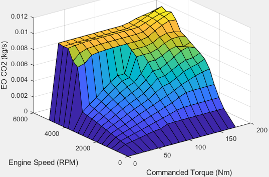## Engine Calibration Maps

Calibration maps are a key part of the Mapped CI Engine and Mapped SI Engine blocks available in the Vehicle Dynamics Blockset™. Engine models use the maps to represent engine behavior and to store optimal control parameters. Using calibration maps in control design leads to flexible, efficient control algorithms and estimators that are suitable for electronic control unit (ECU) implementation.

To develop the calibration maps for engine plant models in the reference applications, MathWorks® developed and used processes to measure performance data from 1.5–L spark-ignition (SI) and compression-ignition (CI) engine models provided by Gamma Technologies LLC.

To represent the behavior of engine plants specific to your application, you can develop your own engine calibration maps. The data required for calibration typically comes from engine dynamometer tests or engine hardware design models.

### Engine Plant Calibration Maps

The engine plant model calibration maps in the Mapped CI Engine and Mapped SI Engine blocks affect the engine response to control inputs (for example, spark timing, throttle position, and cam phasing).

To develop the calibration maps in the engine plant models, MathWorks used GT-POWER models from the GT-SUITE modeling library in a Simulink®-based virtual dynamometer. MathWorks used the Model-Based Calibration Toolbox™ to create design-of-experiment (DoE) test plans. The Simulink-based virtual dynamometer executed the DoE test plan on GT-POWER 1.5–L SI and CI reference engines. MathWorks used the Model-Based Calibration Toolbox to develop the engine plant model calibration maps from the GT-POWER.

#### Calibration Maps in the Mapped CI Engine Block

The Mapped CI Engine block implements these calibration maps.

MapUsed ForInDescription
Engine brake torque

Engine brake torque as a function of commanded fuel mass and engine speed

Mapped CI Engine

The engine brake torque lookup table is a function of commanded fuel mass and engine speed, ${T}_{brake}$ = ƒ(F, N), where:

• ${T}_{brake}$ is engine torque, in N·m.

• F is commanded fuel mass, in mg per injection.

• N is engine speed, in rpm.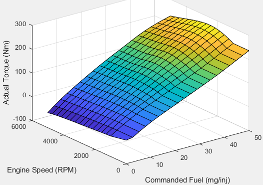Engine air mass flow

Engine air mass flow as a function of commanded fuel mass and engine speed

Mapped CI Engine

The air mass flow lookup table is a function of commanded fuel mass and engine speed, ${\stackrel{˙}{m}}_{intk}$ = ƒ(Fmax, N), where:

• ${\stackrel{˙}{m}}_{intk}$ is engine air mass flow, in kg/s.

• Fmax is commanded fuel mass, in mg per injection.

• N is engine speed, in rpm.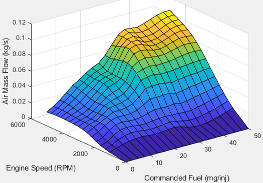Engine fuel flow

Engine fuel flow as a function of commanded fuel mass and engine speed

Mapped CI Engine

The engine fuel flow lookup table is a function of commanded fuel mass and engine speed, MassFlow= ƒ(F, N), where:

• MassFlow is engine fuel mass flow, in kg/s.

• F is commanded fuel mass, in mg per injection.

• N is engine speed, in rpm.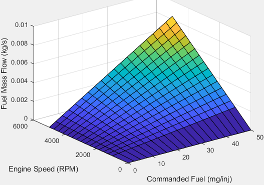Engine exhaust temperature

Engine exhaust temperature as a function of commanded fuel mass and engine speed

Mapped CI Engine

The engine exhaust temperature table is a function of commanded fuel mass and engine speed, Texh= ƒ(F, N), where:

• Texhis exhaust temperature, in K.

• F is commanded fuel mass, in mg per injection.

• N is engine speed, in rpm.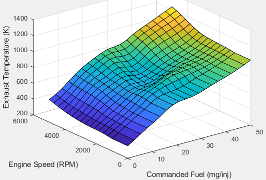Brake-specific fuel consumption (BSFC) efficiency

BSFC efficiency as a function of commanded fuel mass and engine speed

Mapped CI Engine

The brake-specific fuel consumption (BSFC) efficiency is a function of commanded fuel mass and engine speed, BSFC= ƒ(F, N), where:

• BSFC is BSFC, in g/kWh.

• F is commanded fuel mass, in mg per injection.

• N is engine speed, in rpm.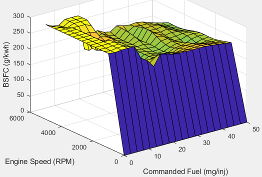Engine-out (EO) hydrocarbon emissions

EO hydrocarbon emissions as a function of commanded fuel mass and engine speed

Mapped CI Engine

The engine-out hydrocarbon emissions are a function of commanded fuel mass and engine speed, EO HC= ƒ(F, N), where:

• EO HC is engine-out hydrocarbon emissions, in kg/s.

• F is commanded fuel mass, in mg per injection.

• N is engine speed, in rpm.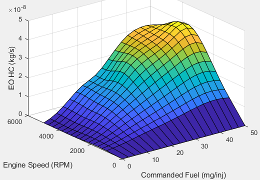Engine-out (EO) carbon monoxide emissions

EO carbon monoxide emissions as a function of commanded fuel mass and engine speed

Mapped CI Engine

The engine-out carbon monoxide emissions are a function of commanded fuel mass and engine speed, EO CO= ƒ(F, N), where:

• EO CO is engine-out carbon monoxide emissions, in kg/s.

• F is commanded fuel mass, in mg per injection.

• N is engine speed, in rpm.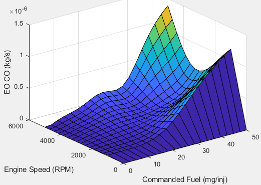Engine-out (EO) nitric oxide and nitrogen dioxide

EO nitric oxide and nitrogen dioxide emissions as a function of commanded fuel mass and engine speed

Mapped CI Engine

The engine-out nitric oxide and nitrogen dioxide emissions are a function of commanded fuel mass and engine speed, EO NOx= ƒ(F, N), where:

• EO NOx is engine-out nitric oxide and nitrogen dioxide emissions, in kg/s.

• F is commanded fuel mass, in mg per injection.

• N is engine speed, in rpm.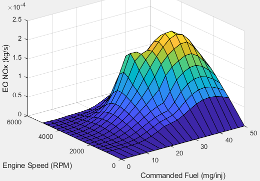Engine-out (EO) carbon dioxide emissions

EO carbon dioxide emissions as a function of commanded fuel mass and engine speed

Mapped CI Engine

The engine-out carbon dioxide emissions are a function of commanded fuel mass and engine speed, EO CO2= ƒ(F, N), where:

• EO CO2 is engine-out carbon dioxide emissions, in kg/s.

• F is commanded fuel mass, in mg per injection.

• N is engine speed, in rpm.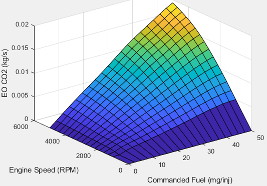#### Calibration Maps in the Mapped SI Engine Block

The Mapped SI Engine block implements these calibration maps.

MapUsed ForInDescription
Engine torque

Engine brake torque as a function of commanded torque and engine speed

Mapped SI Engine

The engine torque lookup table is a function of commanded engine torque and engine speed, T = ƒ(Tcmd, N), where:

• T is engine torque, in N·m.

• Tcmd is commanded engine torque, in N·m.

• N is engine speed, in rpm.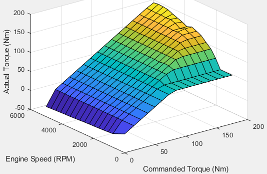Engine air mass flow

Engine air mass flow as a function of commanded torque and engine speed

Mapped SI Engine

The engine air mass flow lookup table is a function of commanded engine torque and engine speed, ${\stackrel{˙}{m}}_{intk}$ = ƒ(Tcmd, N), where:

• ${\stackrel{˙}{m}}_{intk}$ is engine air mass flow, in kg/s.

• Tcmd is commanded engine torque, in N·m.

• N is engine speed, in rpm.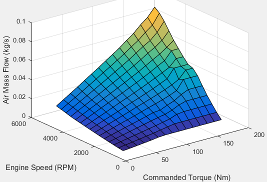Engine fuel flow

Engine fuel flow as a function of commanded torque mass and engine speed

Mapped SI Engine

The engine fuel mass flow lookup table is a function of commanded engine torque and engine speed, MassFlow = ƒ(Tcmd, N), where:

• MassFlow is engine fuel mass flow, in kg/s.

• Tcmd is commanded engine torque, in N·m.

• N is engine speed, in rpm.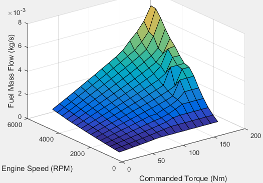Engine exhaust temperature

Engine exhaust temperature as a function of commanded torque and engine speed

Mapped SI Engine

The engine exhaust temperature lookup table is a function of commanded engine torque and engine speed, Texh = ƒ(Tcmd, N), where:

• Texh is exhaust temperature, in K.

• Tcmd is commanded engine torque, in N·m.

• N is engine speed, in rpm.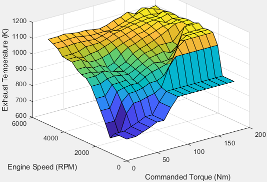Brake-specific fuel consumption (BSFC) efficiency

Brake-specific fuel consumption (BSFC) as a function of commanded torque and engine speed

Mapped SI Engine

The brake-specific fuel consumption (BSFC) efficiency is a function of commanded engine torque and engine speed, BSFC = ƒ(Tcmd, N), where:

• BSFC is BSFC, in g/kWh.

• Tcmd is commanded engine torque, in N·m.

• N is engine speed, in rpm.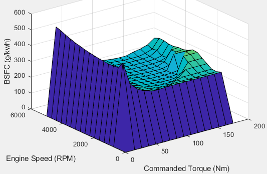Engine-out (EO) hydrocarbon emissions

EO hydrocarbon emissions as a function of commanded torque and engine speed

Mapped SI Engine

The engine-out hydrocarbon emissions are a function of commanded engine torque and engine speed, EO HC = ƒ(Tcmd, N), where:

• EO HC is engine-out hydrocarbon emissions, in kg/s.

• Tcmd is commanded engine torque, in N·m.

• N is engine speed, in rpm.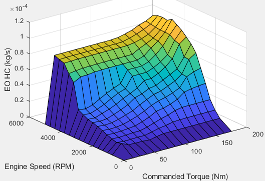Engine-out (EO) carbon monoxide emissions

EO carbon monoxide emissions as a function of commanded torque and engine speed

Mapped SI Engine

The engine-out carbon monoxide emissions are a function of commanded engine torque and engine speed, EO CO = ƒ(Tcmd, N), where:

• EO CO is engine-out carbon monoxide emissions, in kg/s.

• Tcmd is commanded engine torque, in N·m.

• N is engine speed, in rpm.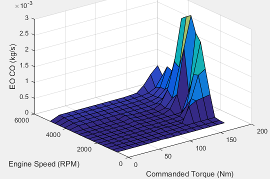Engine-out (EO) nitric oxide and nitrogen dioxide emissions

EO nitric oxide and nitrogen dioxide emissions as a function of commanded torque and engine speed

Mapped SI Engine

The engine-out nitric oxide and nitrogen dioxide emissions are a function of commanded engine torque and engine speed, EO NOx = ƒ(Tcmd, N), where:

• EO NOx is engine-out nitric oxide and nitrogen dioxide emissions, in kg/s.

• Tcmd is commanded engine torque, in N·m.

• N is engine speed, in rpm.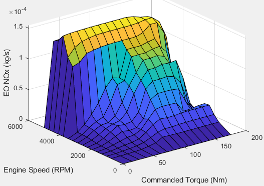Engine-out (EO) carbon dioxide emissions

EO carbon dioxide emissions as a function of commanded torque and engine speed

Mapped SI Engine

The engine-out carbon dioxide emissions are a function of commanded engine torque and engine speed, EO CO2 = ƒ(Tcmd, N), where:

• EO CO2 is engine-out carbon dioxide emissions, in kg/s.

• Tcmd is commanded engine torque, in N·m.

• N is engine speed, in rpm.